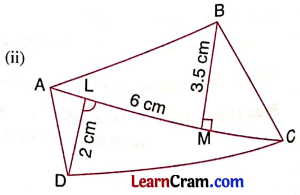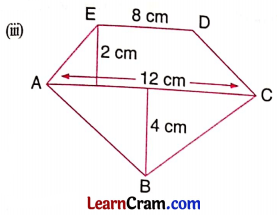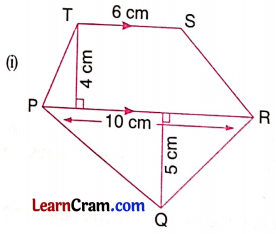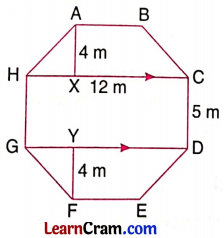# DAV Class 8 Maths Chapter 14 Worksheet 2 Solutions

The DAV Maths Book Class 8 Solutions Pdf and DAV Class 8 Maths Chapter 14 Worksheet 2 Solutions of Mensuration offer comprehensive answers to textbook questions.

## DAV Class 8 Maths Ch 14 WS 2 Solutions

Question 1.
Find the area of the following quadrilaterals:Solution:
(i) Area of quadrilateral PQRS = $$\frac{1}{2}$$ × SQ × (PX + RY)
= $$\frac{1}{2}$$ × 7 × (4 + 3)
= $$\frac{1}{2}$$ × 7 × 7
= $$\frac{49}{2}$$
= 24.5 cm2

(ii) Area of quadrilateral ABCD = $$\frac{1}{2}$$ × AC × (DC + BM)
= $$\frac{1}{2}$$ × 6 × (2 + 3.5)
= $$\frac{1}{2}$$ × 6 × 5.5
= 16.5 cm2

(iii) Area of the polygon ABCDE = ar(∆ABC) + ar(Trapezium CDEA)
= $$\frac{1}{2}$$ × 12 × 4 + $$\frac{1}{2}$$ × (12 + 8) × 2
= 24 + 20
= 44 cm2Question 2.
Find the area of the following polygons:Solution:
(i) Area of the polygon PQRST = ar(∆PQR) + ar(Trapezium PRST)
= $$\frac{1}{2}$$ × 10 × 5 + $$\frac{1}{2}$$ × (10 + 6) × 4
= 25 + 32
= 57 cm2

(ii) Area of the polygon ABCDEF = ar(∆ABF) + ar(∆FBC) + ar(∆FCD) + ar(∆FDE)
= $$\frac{1}{2}$$ × 6.5 × 2 + $$\frac{1}{2}$$ × 7 × 4 + $$\frac{1}{2}$$ × 7 × 4 + $$\frac{1}{2}$$ × 5 × 2
= 6.5 + 14 + 14 + 5
= 39.5 cm2

Question 3.
The top surface of a raised platform is in the shape of a regular octagon as shown in the given figure. Find the area enclosed by an octagonal figure.Solution:
Area of the octagon ABCDEFGH = ar(Trap. ABCH) + ar(HCDG) + ar(Trap. DEFG)
= $$\frac{1}{2}$$ × (12 + 5) × 4 + 12 × 5 + $$\frac{1}{2}$$ × (12 + 5) × 4
= $$\frac{1}{2}$$ × 17 × 4 + 60 + $$\frac{1}{2}$$ × 17 × 4
= 34 + 60 + 34
= 128 cm2Question 4.
There is a pentagonal park. Neha and Nidhi divided it in two different ways to find its area.Find the area of the park in both ways.
Solution:
Neha’s figure:
The given pentagonal figure is divided into two equal trapezium.
∴ Area of ACDEF = 2 × ar(Trap ABEF)
= 2 × $$\frac{1}{2}$$ × (sum of parallel sides) × AB
= 2 × $$\frac{1}{2}$$ × (28 + 15) × 6
= 43 × 6
= 258 cm2

Nidhi’s figure:
The given pentagonal figure is divided into a rectangle ABCE and a ∆DEC.
∴ Area of ABODE = ar(Rectangle ABCE) + ar(∆DEC)
= 12 × 15 + $$\frac{1}{2}$$ × 12 × 13
= 180 + 78
= 258 m2